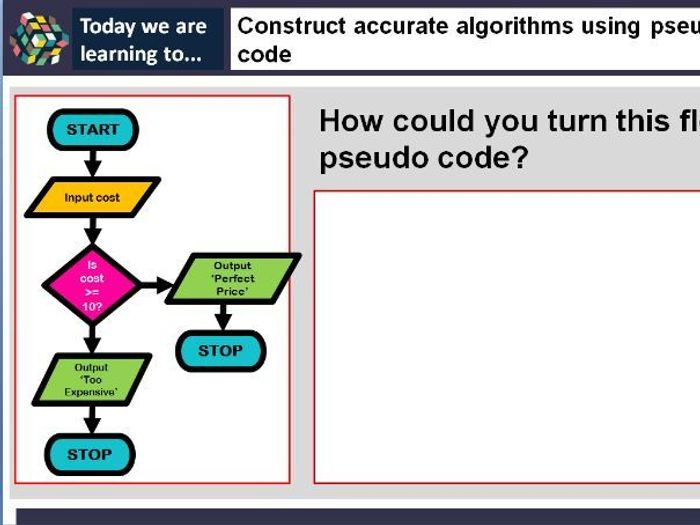Based around an introduction to algorithms, a total of 5 lessons are included in this package.

Aimed at GCSE Computer Science, the 5 lessons are aimed at promoting an introduction to algorithms using decomposition.

Every lesson comes complete with a teachers PowerPoint, iterative starter activities, lesson resources and an ‘Independent Learning Time’ section (this is aimed at allowing students to work independently to consolidate their understanding). Answers to flowchart and pseudo code tasks are included on the PowerPoints.

Lesson 1:
Introduction to algorithms using flowcharts, starts off with basic flowcharts based on easy scenarios and builds up to looking back at sound storage and representing this process as a flowchart.

Lesson 2:
Looking at more complex flowcharts to represent algorithms, focusing more on selection and iteration within algorithms. Independent Learning Activity includes exam questions for students to decompose problems and represent as flowcharts.

Lesson 3:
The focus of this lesson is to allow students time to work independently at solving complex algorithms as flowcharts. Using sequencing, selection and iteration a range of exam questions are included. Class activities are included beforehand to recap the learning of flowcharts.

Lesson 4:
An introduction to pseudo code - links the understanding of what pseudo code is to Python code which students may have come across beforehand from their NEA. Different examples of how code should be constructed are included. Several flowcharts are included on the PowerPoint which students then need to turn into pseudo code. Sequencing and selection are focused upon in this lesson.

Lesson 5:
Developing students understanding further using sequencing, selection and iteration. WHILE loops are demonstrated of how to construct a loop in pseudo code. Students will spend their time working on problems and trying to decompose these into pseudo code. Extension questions are included for the HAL students / students needing extra or more challenging work.

£4.00

### Info

Created: Apr 17, 2019

zip, 4 MB

Algorithms

Report a problem

### Tes Paid Licence

How can I re-use this?